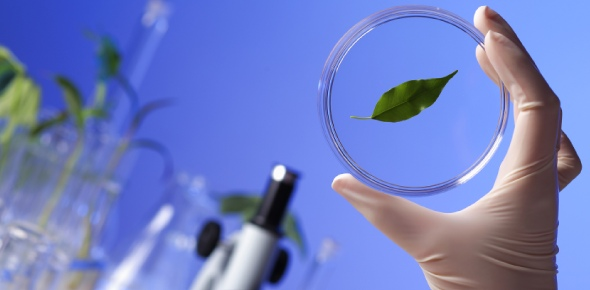# Biology I Final Exam (Practice)

90 Questions | Total Attempts: 225SettingsCreate your own QuizI took all of the questions from past quizzes in Biology and compiled them all onto one test. Hopefully this will help prepare for the final exam. This does not include the open discussion quizzes that was on the exam.

• 1.
Which sequence correctly lists the different levels of biological organization?
• A.

A. cells -> organs -> tissues -> organ system -> organism

• B.

B. cells -> tissues -> organ system -> organs -> organism

• C.

C. tissues -> cells -> organ -> organ system -> organism

• D.

D. tissues -> organs -> organ system -> organisms -> cells

• E.

E. cells -> tissues -> organs -> organ system -> organism

• 2.
Characteristics of life include all BUT
• A.

A. growth and development

• B.

B. reproduction

• C.

C. energy use

• D.

D. mimicry

• 3.
Domains or "superkingdoms" include all BUT
• A.

A. Bacteria

• B.

B. Archaea

• C.

C. Eukarya

• D.

D. Animalia

• 4.
Charles Darwin proposed the theory of evolution by the mechanism of ____________.
• A.

A. mimicry

• B.

B. natural selection

• C.

• D.

D. discovery

• 5.
Principles of a theory include all BUT
• A.

A. body of interconnected concepts

• B.

B. expresses ideas of which we are most certain

• C.

C. explains natural phenomen

• D.

D. supported by much experimental evidence

• 6.
The atomic weight of an element is calculated by
• A.

A. number of protons

• B.

B. number of neutrons

• C.

C. number of protons + neutrons

• D.

D. number of protons + electrons

• 7.
Covalent bonding is described as
• A.

A. sharing of electrons

• B.

B. sharing of protons

• C.

C. loss or gain of electrons

• D.

D.loss or gain of neutrons

• 8.
How many electrons is required for an element to be stable, in essence non-reactive
• A.

A. six

• B.

B. seven

• C.

C. eight

• D.

D. nine

• 9.
Polar molecules are generated by
• A.

A. ionic bonding

• B.

B. covalent bonding

• C.

C. cations

• D.

D. anions

• 10.
As air temperaturewarms duringthe day from 50 to 70 degrees, water in the same area will
• A.

A. warm the same as air

• B.

B. warm more quickly than air

• C.

C. warm more slowly

• D.

D. not change at all in temperature

• 11.
Organic compounds are categorized into four classes including all EXCEPT
• A.

A. water

• B.

B. lipids

• C.

C. proteins

• D.

D. nucleic acids

• E.

E. carbohydrates

• 12.
Features of the element, carbon, include all BUT
• A.

A. forms covalent bonds

• B.

B. has 4 electrons in its outer shell (valence)

• C.

C. along with hydrogen, included in all organics

• D.

D. forms ionic bonds

• 13.
Lipids include all BUT
• A.

A. phospholipids

• B.

B. albumin

• C.

C. waxes

• D.

D. steroids

• 14.
Ose is a common suffix with which type of compounds?
• A.

A. sugars

• B.

B. steroids

• C.

C. proteins

• D.

D. acids and bases

• E.

E. nucleic acids

• 15.
What characteristic of a protein will determine its function?
• A.

A. pH level

• B.

B. shape

• C.

C. location

• D.

D. length

• 16.
Which association is NOT correct?
• A.

A. nucleic acid - RNA or DNA

• B.

B. carbohydrates - enzymes

• C.

C. fats - long term storage of energy

• D.

D. proteins - structures

• E.

E. cellulose - plant cell wall

• 17.
Which of the following DOES NOT apply to carbohydrates?
• A.

A. may be monsaccharides, disaccharides, or polysaccharides

• B.

B. complex include starches and glycogen

• C.

C. may be saturated or unsaturated

• D.

D. composed of carbon, hydrogen, and oxygen atoms

• 18.
A nucleotide consists of
• A.

A. double helix

• B.

B. a sugar, a nitrogenous base, and a phosphate group

• C.

C. only carbon and hydrogen atoms

• D.

D. only within DNA

• 19.
Appropriate descriptions for a plasma mebrane include all BUT
• A.

A. fluid

• B.

B. rigid

• C.

C. mosiac

• D.

D. selectively permeable

• 20.
Types of passive transport include all BUT
• A.

A. diffusion

• B.

B. facilitated diffusion

• C.

C. receptor-mediated

• D.

D. osmosis

• 21.
A solution that has a higher concentration of solute and a lower concentration of water than inside the cell is
• A.

A. isotonic solution

• B.

B. hypotonic solution

• C.

C. hypertonic solution

• D.

D.osmotic solution

• 22.
Forms of endocytosis include all BUT
• A.

A. exocytosis

• B.

B. phagocytosis

• C.

C. pinocytosis

• D.

D. receptor-mediated

• 23.
An isotonic solution means that the solute concentraion outside the cell is
• A.

A. is the same as inside the cell

• B.

B. is less than inside the cell

• C.

C. is greater than inside the cell

• D.

D. has no effect on the cell

• 24.
Which of thefollowing is (are) true about facilitated diffusion?
• A.

A. requires a concentration gradient

• B.

B. requires a membrane

• C.

C. involves proteins

• D.

D. doesn't involve an outside energy source

• E.

E.all of the above

• 25.
White blood cells engulf bacteria through what process
• A.

A. exocytosis

• B.

B. phagocytosis

• C.

C. pinocytosis

• D.

D. osmosis

• E.

E. receptor-mediated exocytosis

Related TopicsBack to top
×

Wait!
Here's an interesting quiz for you.